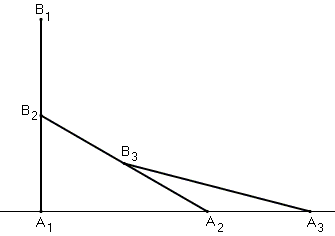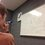# The Golden Ratio: The Most Elegant ConstructionHere is the previous post concerning the Golden Ratio. For a collection of all the posts concerning the Golden Ratio, click #GoldenRatio below.

Sorry for the long wait! This is the final post about the geometry of the golden ratio, and to celebrate that, I will present to you, with proof, the most elegant construction of the golden ratio. This construction is so awesome, it can even be performed without a compass, paper, or pencil.

CONSTRUCTION:

Take three segments of equal length (whether they be toothpicks, playing cards, or a drawing on a piece of paper). Stand one upright perpendicular to a flat surface. Now place the next one so that it touches the flat surface and the midpoint of the other segment. Place the final one so that it touches the flat surface and the midpoint of the middle segment. The line formed on the flat surface is divided into the golden ratio. See the above picture.

PROOF:

Let the segments have length 2. Then $A_1B_2 = 1$, making $A_1A_2 = \sqrt{2^2-1^2} = \sqrt{3}$. Draw the perpendicular from $B_3$ to the surface. It has length $\frac{1}{2}$. Then $A_2A_3 = \sqrt{2^2 - \left(\frac{1}{2}\right)^2} - \sqrt{1^2 - \left(\frac{1}{2}\right)^2} = \sqrt{\frac{15}{4}} - \sqrt{\frac{3}{4}} = \sqrt{3} \frac{\sqrt{5}-1}{2}$. Then we can take the ratio:

$\frac{A_1A_2}{A_2A_3} = \frac{\sqrt{3}}{\sqrt{3} \frac{\sqrt{5}-1}{2}} = \frac{1+\sqrt{5}}{2} = \phi$

Be prepared for an even more exciting quest into the algebraic mysterious of the golden ratio!

NextNote by Bob Krueger
6 years, 4 months ago

This discussion board is a place to discuss our Daily Challenges and the math and science related to those challenges. Explanations are more than just a solution — they should explain the steps and thinking strategies that you used to obtain the solution. Comments should further the discussion of math and science.

When posting on Brilliant:

• Use the emojis to react to an explanation, whether you're congratulating a job well done , or just really confused .
• Ask specific questions about the challenge or the steps in somebody's explanation. Well-posed questions can add a lot to the discussion, but posting "I don't understand!" doesn't help anyone.
• Try to contribute something new to the discussion, whether it is an extension, generalization or other idea related to the challenge.

MarkdownAppears as
*italics* or _italics_ italics
**bold** or __bold__ bold
- bulleted- list
• bulleted
• list
1. numbered2. list
1. numbered
2. list
Note: you must add a full line of space before and after lists for them to show up correctly
paragraph 1paragraph 2

paragraph 1

paragraph 2

[example link](https://brilliant.org)example link
> This is a quote
This is a quote
    # I indented these lines
# 4 spaces, and now they show
# up as a code block.

print "hello world"
# I indented these lines
# 4 spaces, and now they show
# up as a code block.

print "hello world"
MathAppears as
Remember to wrap math in $$ ... $$ or $ ... $ to ensure proper formatting.
2 \times 3 $2 \times 3$
2^{34} $2^{34}$
a_{i-1} $a_{i-1}$
\frac{2}{3} $\frac{2}{3}$
\sqrt{2} $\sqrt{2}$
\sum_{i=1}^3 $\sum_{i=1}^3$
\sin \theta $\sin \theta$
\boxed{123} $\boxed{123}$

Sort by:

That is AWESOME!!!!!

- 6 years, 4 months ago